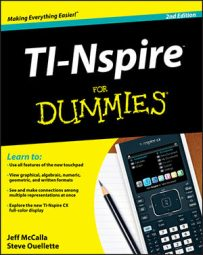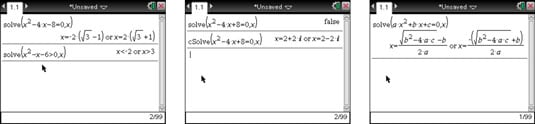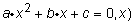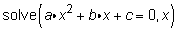##### TI-Nspire For DummiesThe TI-Nspire CAS capabilities found in the Algebra submenu are nothing short of amazing. The Solve command returns the solution(s) to an equation or inequality. Choose [MENU]→Algebra→Solve to open the Solve command.Using the Solve and cSolve commands.

The first screen shows how the Solve command can solve a single equation or an inequality, with solutions displayed in symbolic form.

The first line in the second screen shows the result of using the Solve command on equations with no real number solutions. The equation x2 – 4x + 8 = 0 has no real number solutions. However, it does have two complex solutions.

To find complex solutions, press [MENU]→Algebra→Complex→Solve. This command, denoted cSolve, returns the complex solutions x = 2 + 2i and x = 2 – 2i for this equation.

Try solving the equationfor the variable x by typingTI-Nspire CAS returns the quadratic formula! Make sure that you press [x] between variables. Otherwise, TI-Nspire CAS may mistakenly think that ax and bx are single variables. See the third screen.